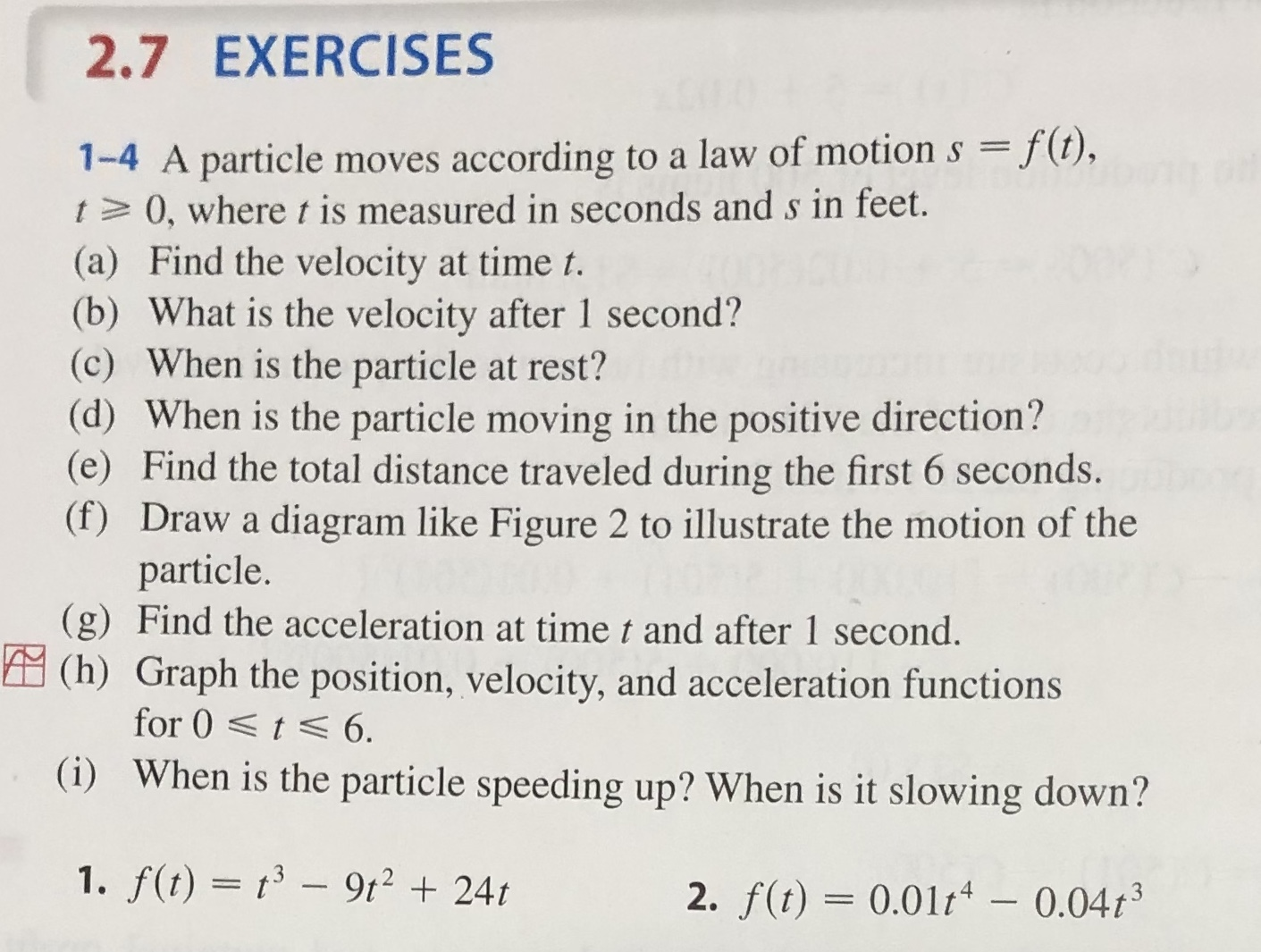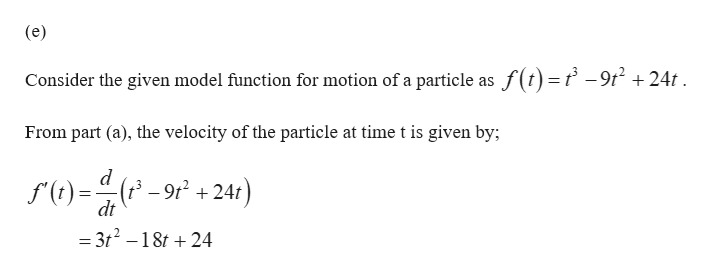# 2.7 EXERCISES1-4 A particle moves according to a law of motion s f(t),t 0, where t is measured in seconds ands in feet.(a) Find the velocity at time t.(b) What is the velocity after 1 second?(c) When is the particle at rest?(d) When is the particle moving in the positive direction?(e) Find the total distance traveled during the first 6 seconds.(f) Draw a diagram like Figure 2 to illustrate the motion of theparticle.(g) Find the acceleration at time t and after 1 second.(h) Graph the position, velocity, and acceleration functionsfor 0t 6.(i) When is the particle speeding up? When is it slowing down?1. f(t) t3-9t224t2. f(t) 0.01t - 0.04t3

Question

2.7

Can you show me the steps to solve step e?

1) f(t) = t^3 - 9t^2 +24t

e) Find the total distance travelled during the first 6 seconds.help_outlineImage Transcriptionclose2.7 EXERCISES 1-4 A particle moves according to a law of motion s f(t), t 0, where t is measured in seconds ands in feet. (a) Find the velocity at time t. (b) What is the velocity after 1 second? (c) When is the particle at rest? (d) When is the particle moving in the positive direction? (e) Find the total distance traveled during the first 6 seconds. (f) Draw a diagram like Figure 2 to illustrate the motion of the particle. (g) Find the acceleration at time t and after 1 second. (h) Graph the position, velocity, and acceleration functions for 0t 6. (i) When is the particle speeding up? When is it slowing down? 1. f(t) t3-9t224t 2. f(t) 0.01t - 0.04t3 fullscreen
check_circleExpert Solution
Step 1help_outlineImage Transcriptionclose(e) Consider the given model function for motion of a particle as f(t)=f -9t2 +24t From part (a), the velocity of the particle at time t is given by; d (t-9r224t dt = 3t2-18t 24 fullscreen

### Want to see the full answer?

See Solution

#### Want to see this answer and more?

Solutions are written by subject experts who are available 24/7. Questions are typically answered within 1 hour*

See Solution
*Response times may vary by subject and question
Tagged in

### Other